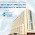Friday, April 3, 2009

The period between 500 and 1200 AD was the golden age of Indian Astronomy. In this long span of time Indian Astronomy flourished mainly due to eminent astronomers like Aryabhatta, Lallacharya, Varahamihir, Brahmagupta, Bhaskaracharya and others. Bhaskaracharya, or Bhaskara II (1114 – 1185) is regarded almost without question as the greatest Hindu mathematician of all time and his contribution to not just Indian, but world mathematics is undeniable. He was born near Bijjada Bida (in present day Bijapur district, Karnataka state, South India) into the Deshastha Brahmin family. Bhaskara was head of an astronomical observatory at Ujjain, the leading mathematical centre of ancient India. His father Maheshwar taught him mathematics and astronomy.

Some of Bhaskara's contributions to mathematics include the following:

• Bhaskara is the first to give the general solution to the quadratic equation ax2 + bx + c = 0, the answer being x = (-b ± (b2 - 4ac)1/2)/2a.
• He also gives the (now) well known results for sin (a + b) and sin (a - b).
• Stated Rolle's Theorem, a special case of one of the most important theorems in analysis, the mean value theorem. Traces of the general mean value theorem are also found in his works.
• Solutions of indeterminate quadratic equations (of the type ax² + b = y²).
• Bhaskara also goes deeper into the 'differential calculus' and suggests the differential coefficient vanishes at an extremum value of the function, indicating knowledge of the concept of 'infinitesimals'

Bhaskaracharya wrote Siddhanta Shiromani in 1150 AD when he was 36 years old. This is a mammoth work containing about 1450 verses. It is divided into four parts and each part can be considered as separate book. The numbers of verses in each part are as follows, 1. Lilawati has 278 2. Beejaganit has 213 3. Ganitadhyaya has 451 4. Goladhyaya has 501 verses.

One of the most important characteristic of Siddhanta Shiromani is, it consists of simple methods of calculations from Arithmetic to Astronomy. Essential knowledge of ancient Indian Astronomy can be acquired by reading only this book. Siddhanta Shiromani has surpassed all the ancient books on astronomy in India. After Bhaskaracharya nobody could write excellent books on mathematics and astronomy in lucid language in India.

Lilawati is an excellent example of how a difficult subject like mathematics can be written in poetic language. Lilawati has been translated in many languages throughout the world. Lilavati covers the topics of definitions, arithmetical terms, interest computation, arithmetical and geometrical progressions, plane geometry, solid geometry. Bhaskara's method of solving was an improvement of the methods found in the work of Aryabhata and subsequent mathematicians.

His work Bijaganita is effectively a treatise on algebra and contains the following topics: - Positive and negative numbers.
- Zero. - The 'unknown'.
- Surds.
- Kuttaka (modern indeterminate equation of first order)
- Simple equations (indeterminate of second, third and fourth degree).
- Simple equations with more than one unknown.
- Indeterminate quadratic equations (of the type ax2 + b = y2).
- Quadratic equations with more than one unknown.
- Operations with products of several unknowns.

Ganitadhyaya and Goladhyaya of Siddhanta Shiromani are devoted to astronomy. All put together there are about 1000 verses. Almost all aspects of astronomy are considered in these two books. Bhaskara has given a very simple method to determine the circumference of the Earth. According to this method, first find out the distance between two places, which are on the same longitude. Then find the correct latitudes of those two places and difference between the latitudes. Knowing the distance between two latitudes, the distance that corresponds to 360 degrees can be easily found, which the circumference of the Earth.

He also showed that when a planet is farthest from, or closest to, the Sun, the difference between a planet's actual position and its position according to "the equation of the centre" (which predicts planets' positions on the assumption that planets move uniformly around the Sun) vanishes. He therefore concluded that for some intermediate position the differential of the equation of the centre is equal to zero.

A Glance at the Astronomical Achievements of Bhaskaracharya

1. The Earth is not flat, has no support and has a power of attraction.

2. The north and south poles of the Earth experience six months of day and six months of night.

3. One day of Moon is equivalent to 15 earth-days and one night is also equivalent to 15 earth-days.

4. Bhaskaracharya had accurately calculated apparent orbital periods of the Sun and orbital periods of Mercury, Venus, and Mars. There is slight difference between the orbital periods he calculated for Jupiter and Saturn and the corresponding modern values.

5. Earth’s atmosphere extends to 96 kilometers and has seven parts.

6. There is a vacuum beyond the Earth’s atmosphere.

There have been several unscrupulous attempts to argue that there are traces of Diophantine influence in Bhaskara's work, but this once again seems like an attempt by European scholars to claim European influence on (all) the great works of mathematics. These claims should be ignored. Particularly in the field of algebra, Diophantus only looked at specific cases and did not achieve the general methods of the Indians.

Related article "Aryabhatta and Evolution of Zero"

1 comment:

1.We are urgently in need of kidney donors in Kokilaben Hospital India for the sum of \$500,000,00,All donors should reply via Email only: kokilabendhirubhaihospital@gmail.com
WhatsApp +91 779-583-3215